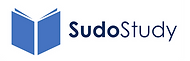top of page

#### Energy

How energy can be stored and the equations for calculating it.

Anchor 1

### 1. Work Done

The work done on an object by an applied force is equal to the force multiplied by the distance moved in the direction of the force. Sometimes the force is applied at an angle so this must be taken into consideration as well.

Anchor 2

### 2. Kinetic Energy

The kinetic energy of an object is the energy it posses by virtue of its motion. In this video (with a little bit of stop frame animation) I also go through the derivation and where there is a v squared term in the equation.

Anchor 3

### 3. Gravitational Potential Energy

Gravitational potential energy is the energy an object has due to its position in a gravitational field, so it depends on the mass, the height and also the gravitational field strength 'g'.

Anchor 4

### 4. Conservation of Mechanical Energy

Energy cannot be created or destroyed - just transferred from one form to another. This is really useful when we consider the change from KE to PE or PE to KE.Anchor 5

### 5. Conservation of Energy

Energy cannot be created or destroyed, just transferred to other stores.bottom of page